## research papersJOURNAL OFAPPLIEDCRYSTALLOGRAPHY
ISSN: 1600-5767
Volume 38| Part 1| February 2005| Pages 193-198

## Likelihood weighting of partial structure factors using spline coefficients

aDepartment of Chemistry, University of York, Heslington, York YO10 5DD, UK
*Correspondence e-mail: cowtan@ysbl.york.ac.uk

(Received 28 June 2004; accepted 29 November 2004)

A method for the weighting of structure factors from an incomplete and inaccurate model is described which relies on the fitting of smooth spline functions of resolution. The use of smooth spline functions avoids the problems of discontinuities introduced when performing calculations in resolution shells. The complexity of the functions to be fit may be varied by changing the number of spline parameters. This approach is used to investigate the stability of the problem when data are limited.

### 1. Background

The task of weighting information arising from an incomplete and inaccurate model of the scattering density of a crystal cell is a vital part of the structure solution and refinement process. Incomplete and inaccurate models arise at all stages of the structure solution process, starting with the crude models arising from molecular replacement or initial density interpretation, right through to refined models which lack only in their description of subtle features such as disorder and bulk solvent. The weighting of incomplete and inaccurate model phases allows calculation of improved maps with which errors and omissions in an existing model can be corrected, and also provides the basis for calculation of difference maps used in the refinement of such models.

An early approach to this problem was proposed by Sim (1959), who assumed that the model was incomplete but without errors. This assumption leads to the result that the measured structure factor must arise from the known partial structure factor plus some unknown component arising from the missing density. If the missing atoms are uniformly distributed, this component will have a uniformly distributed phase and a magnitude which varies according to the amount of un-modelled density. Sim proposed that the unknown part should obey a circular Gaussian distribution in the Argand diagram, the width of which was determined by the disagreement between the observed and partial structure-factor magnitudes (or lack-of-closure'). This Gaussian distribution may be projected on to the circle described by the observed structure-factor magnitude, giving rise to a phase probability distribution centred about the phase of the partial structure factor.

Srinivasan & Ramachandran (1965) developed a theoretical framework to extend this approach to incorporate the effect of errors in the model into the calculation based on the parameter σa, which combines information about model completeness and accuracy. They noted that model errors on average reduce the magnitude of the correct part of the partial structure factor and add an additional contribution to the Gaussian error term. Read (1986) implemented this approach by constructing a likelihood function which gave the probability of the observed structure-factor magnitudes given a particular combination of model incompleteness and error. The values of σa that maximize this probability across all reflections provide a better basis for weighting the resulting phases.

A formalized treatment of this work in terms of likelihood is given by Murshudov et al. (1997). This approach has also been extended to the case where some phase information is known, by Pannu et al. (1998). A related description is given by Lunin et al. (2002).

Read (1986) originally calculated σa in resolution bins. The available reflections were divided up into shells in reciprocal space, with each shell covering a narrow resolution range. The likelihood function was maximized to determine the value of σa for each shell individually, to allow σa to vary as a function of resolution. About 1000 reflections are required in each resolution shell to obtain a reliable estimate of σa.

Later it became desirable to calculate σa using the free-set reflections alone (Brünger, 1993) to avoid the value being biased by previous cycles of model refinement. This was accomplished by Murshudov et al. (1997) by abandoning the use of resolution bins, and instead fitting σa using a continuous function of resolution. Murshudov et al. (1997) uses a form composed of two interacting Gaussian terms originally proposed by Tronrud (1997) as a Babinet correction for un-modelled bulk-solvent contributions. This approach allowed σa to be estimated using as few as 200 reflections, although a sophisticated minimizer is required to arrive at that solution.

This paper identifies a simple approach, which is applicable when an intermediate number of reflections are available, by which parameters related to σa may be modelled using spline functions of an arbitrary number of reflections. This was attempted by Cowtan (2002); however, the method used there suffered from stability problems. This work overcomes those problems by choosing a different parameterization in which two parameters are modelled simultaneously. These parameters are a lack-of-closure parameter and a scale factor, which both scales the calculated data to the observed data and reduces the calculated magnitudes to account for errors in the calculated phase.

### 2. Method

Murshudov gives the likelihood function for the magnitude of an observed structure factor given some partial structure factor as follows (Murshudov et al., 1997, equation 14 therein):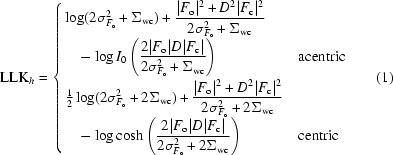In these equations,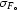is the experimental error in the observed magnitude, D is the proportion of the calculated structure factor which is correct and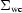is the variance of the Gaussian error term. Constants have been ignored, and the equations have been simplified for a single partial structure. An extra factor of 2 has been introduced inside the logarithm in the centric term; this is for later convenience and is cancelled by an additional contribution to the (omitted) constant term.

The equations may be further simplified by substituting=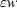, and definingas the reflection multiplicity, given by the number of symmetry operators relating the reflection to itself, and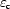as the number of symmetry operators relating a reflection to itself or its Friedel opposite: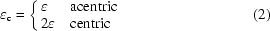This gives a single equation for centric and acentric cases: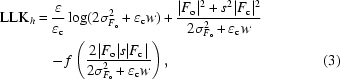where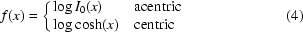Here w scales the width of the Gaussian error term. s plays an identical role to D in the original equations, but will also take into account any difference in scale between the observed and calculated data. These parameters are very similar to the α and β of Lunin et al. (2002), with the latter differing by a factor of 2 in the centric case.

The first and second derivatives of this function may be constructed with respect to s and w.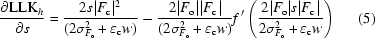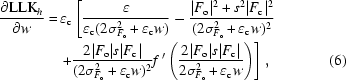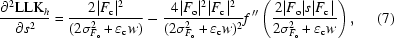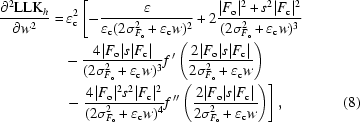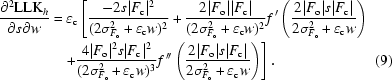The complete log-likelihood is obtained by summing the individual reflection log-likelihoods (i.e. multiplying the probabilities) over all the reflections (e.g. Murshudov et al., 1997, equation 13 therein).

For this work, we will assume that the variables s and w vary continuously as a function of resolution [i.e. s = s(|h|), w = w(|h|)], according to some previously chosen function which depends on some numerical parameters. Thus s and w are each described by a basis function', using the terminology of Cowtan (2002). For this paper, this basis function is assumed to be a spline function of resolution as described by Cowtan (2002); however the approach is independent of the choice of this function.

The derivatives of the individual reflection log-likelihood LLKh with respect to s(|h|) and w(|h|) may be derived and combined with the derivatives of the basis function with respect to its parameters (e.g. Cowtan, 2002,equations 10 and 13 therein) using the chain rule in order to construct the derivatives of the log-likelihood function with respect to the basis function parameters. Summing the derivatives over all reflections gives rise to the derivatives of the full log-likelihood function. The resulting derivatives may be used in a Newton–Raphson calculation to iteratively determine the optimal values of the basis function parameters.

Once the optimal parameters of s and w are obtained as a function of resolution, a figure-of-merit may be calculated for each reflection, according to the formula: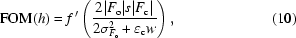where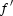(X) is the derivative of f(X), which is I1(X)/I0(X)for acentric reflections and tanh(X) for centric reflections.

### 3. Implementation

The spline function of resolution was implemented as part of earlier work on fitting arbitrary functions in reciprocal space, and that code was re-used in this work. The remaining code from that work was specialized to single-valued functions of position, whereas in this case two functions are used, for s and w, and so this code was not reused. Instead the target function was implemented, returning LLKh and its first two derivatives with respect to s(h) and w(h). This was used in conjunction with spline basis functions for s and w, in a purpose-written routine which constructed LLK and its derivatives with respect to the two sets of spline parameters using the chain rule. These derivatives were then used to implement a simple Newton–Raphson optimization to determine the best parameters.

In early tests the full curvature matrix was calculated, but it was found that the cross terms linking the parameters of s and w, while helpful during the final stages of parameter refinement, are a hindrance in the earlier stages of refinement. It is therefore more efficient to break the matrix of curvatures into two diagonal N × N blocks containing the curvatures relating the s and w parameters, respectively. The shifts to the s and w parameters may then be determined by solving two N × N series of equations, instead of one 2N × 2N system.

One additional refinement was required in order to solve two problems. Firstly, spline coefficients are prone to oscillations when they are used to fit sharply varying functions. These oscillations are analogous to the ripples found in Fourier series of discontinuous functions. As a result it is beneficial to pre-scale the data in such a way as to remove the most steeply varying features. Secondly, for the Newton–Raphson calculation to converge, a reasonable estimate of the parameters is required as a starting point. This is also achieved as a side-effect of pre-scaling.

The data (both Fo and Fc are therefore scaled onto an approximate E-like scale, by dividing each structure factor by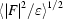, where the expectation value is again obtained by a spline fit to the data. The resulting scaled magnitudes are not, however, Es, because the effect of the reflection multiplicity has not been removed. Furthermore no assumption is made about the correctness of this scaling in the remainder of the calculation.

Since both Fo and Fc are now on roughly the same scale, an initial estimate of s may be made by setting all the spline coefficients, and thus the value of the function itself, to 1. Since w is to a first approximation related to the squared difference between |Fo| and |Fc|, and these are on a roughly |E|-like scale, this may also be set to 1 as a crude order-of-magnitude estimate. Using these estimates, the calculation converges reliably in between 5 and 15 cycles. The results are robust against different initial estimates, even for poor models. However, as will be shown, the results may be sensitive to the choice of data.

### 4. Results

Initial tests were conducted by comparing the results of this implementation with a version of the original implementation of Read (1986), as modified and distributed as part of the CCP4 suite (Collaborative Computational Project, Number 4, 1994). A simulated molecular replacement was constructed using the observed data for the lysozyme structure 1lz8 (Dauter et al., 1999), which was obtained from the Protein Data Bank (Berman et al., 2000). Calculated data were obtained from the atomic model of 1hhl (Lescar et al., 1994), which was rotated and translated to match the target structure. The model was truncated to include only main chain and Cβ atoms, and structure factors were calculated using refmac5 (Murshudov et al., 1997), with no bulk-solvent correction.

Figures-of-merit were calculated using the original binned σa approach, and using the spline fit for s and w, using all the available reflections. The mean figure-of-merit was calculated in resolution bins and plotted as a function of resolution for both calculations in Fig. 1. The features of this plot depend both on the estimates of σa or s and w, and on the observed and calculated magnitudes in each resolution shell. Note that over most of the resolution range the mean figure-of-merit agrees well between the two calculations. The largest deviations between the two calculations arise at the low resolution end, where the discontinuities arising from bin boundaries will be most pronounced. The agreement confirms firstly that the approach is working, and secondly that the effect of the experimental, which is omitted in Read's original implementation, does not have a significant impact in this case.Figure 1 Comparison of mean figure-of-merit as a function of resolution between different implementations of the weighting calculation. The program of Read (1986) is compared with the new spline-based implementation.

It is important to determine how many reflections are required to obtain reliable weight estimates using a given number of spline parameters in the fitting of s and w, so that a suitable functional form may be chosen for any given problem. This is particularly important for refinement calculations where s and w may be determined using the free-set reflections only. For this purpose the data for a larger glycosidase structure, 1h2j (Varrot & Davies, 2003), were used.

Again molecular-replacement type models were constructed using related structures from the PDB. Three models of decreasing quality were used: an all-atom model of 1h11 (Varrot & Davies, 2003), a main chain + Cβ model of 1qi2 (Varrot et al., 2000) and a main chain + Cβ model of 1g0c (Shirai et al., 2001). The coordinate errors of these models are listed in Table 1. The models were aligned using the SSM secondary structure alignment method (Krissinel & Henrick, 2004).

 Table 1Simulated molecular replacement models used in testing the structure-factor weighting algorithm
Model Cα r.m.s.d. (Å) Completeness R factor
1h11 0.16 All atoms 0.324
1qi2 0.37 Main chain + Cβ 0.436
1g0c 0.99 Main chain + Cβ 0.537

The original data for 1h2j included ∼47500 reflections to 1.5 Å resolution. These data were divided into first 20 sets of ∼2500 reflections and then five sets of ∼10000 reflections. Weighting calculations were performed using the reflections from each of five different free sets in each case to fit spline functions to s and w. The results using each set of reflections were compared to determine how many reflections are required for a particular parameterization.

Initially phase weighting calculations were performed using the intermediate model, 1qi2, using free sets of ∼2500 reflections. These calculations were performed using different numbers of spline parameters. Comparisons of the resulting mean figure-of-merit as a function of resolution are shown in Figs. 2and 3for the results of using three spline parameters and 15 spline parameters. When only three spline parameters are fitted, the results are very similar whichever free set is used; however, when 15 spline parameters are used, the variation in mean figure-of-merit can be substantial. For subsequent tests it will be assumed that the first of these results represents the limit of acceptable deviation between free sets.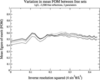Figure 2 Comparison of mean figure-of-merit as a function of resolution between model weighting calculations using different free sets and three spline parameters.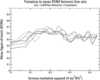Figure 3 Comparison of mean figure-of-merit as a function of resolution between model weighting calculations using different free sets and 15 spline parameters.

In Fig. 2it is possible to see the contribution of observed and calculated magnitudes to the figure-of-merit. Since only three spline parameters are used, s and w may have only three extrema, and the remaining features of this plot arise from the individual structure factors, which are conserved between different estimates of s and w. In Fig. 3, s and w may have as many as 15 extrema and the features vary between estimates, so the errors in s and w are responsible for the bulk of the features.

To quantify these results for further analysis, the standard deviation of the figures-of-merit among the five sampled free sets was calculated for each model, parameterization and choice of free reflections. The results of these calculations are shown in Figs. 4, 5and 6. Note that the adequate result in Fig. 2corresponds to an FOM standard deviation of about 0.017, so a deviation of greater than 0.02 will be considered unacceptable.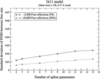Figure 4 Standard deviation between figures-of-merit using different free sets as a function of number of parameters for a complete and accurate model (1h11).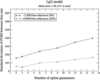Figure 5 Standard deviation between figures-of-merit using different free sets as a function of number of parameters for a partial (main chain + Cβ) and intermediate model (1qi2).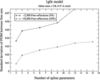Figure 6 Standard deviation between figures-of-merit using different free sets as a function of number of parameters for a partial (main chain + Cβ) and poor model (1g0c).

For the best model, 1h11, Fig. 4shows that with 10000 reflections it is possible to fit 15 or more spline parameters without difficulty. With only 2500 free reflections, nine parameters can be fitted reliably. Thus in the case of a good model, such as a model in the final stages of refinement, only 250 reflections are required per spline parameter. This is more parameters than are required for the smooth function used by Murshudov et al. (1997), but is usable for the refinement of medium and large macromolecules.

For the intermediate model, 1qi2, Fig. 5shows that with 10000 reflections it is possible to fit 15 or more parameters; however, with only 2500 reflections only three parameters may be reliably determined. This corresponds roughly to the case of an incomplete model in the early stages of refinement. In this case around 750 reflections are required for each spline parameter. Again this is usable for medium or large macromolecules as long as only a few spline parameters are used in the early stages of refinement.

For the worst model, 1g0c, Fig. 6shows that with 2500 reflections no reliable results are obtained. With 10000 reflections, it is possible to fit two or three spline parameters. This corresponds roughly to the case of a poor first molecular replacement model, before initial rebuilding. The quality of the phasing is also not dissimilar to that which arises in some density modification calculations (see for example Abrahams, 1997). In this case it would be wise to use all the reflections in the estimation of the spline parameters, and to use a limited number of spline parameters. At this stage of the calculation model bias will be far less of an issue since no refinement of the model has taken place, and so the use of all the reflections is not a major problem.

Comparisons were made between weighted maps obtained using the centroid weights obtained using the binned and spline approaches using different numbers of parameters. Initially the new method was thought to give more reliable results when only a few parameters were used; however, it was later found that the main difference arose from the spline scaling of the data, rather than spline fitting of the s and w functions. Once this effect was removed, the resulting maps were effectively indistinguishable between old and new methods and different numbers of parameters. The benefits of the spline approach may be more significant for density modification calculations, where resolution cutoffs of isomorphous derivative phasing may yield less smoothly varying values for s and w.

### 5. Conclusions

The method presented here provides a simple means for the weighting of phase information from incomplete and inaccurate models using a continuous function of resolution. This approach offers the theoretical benefit over the use of resolution bins that there are no discontinuities at bin boundaries, which will be particularly pronounced when the number of bins are small. Unlike the previous technique of using a continuous function for phase weighting (Murshudov et al., 1997), the complexity of the functions used here may be varied in accordance with the number of data available and the amount of information present in that data.

This has led to the investigation of the reliability of the result as a function of number of data and quality of the model. The data-to-parameter ratio is shown to vary strongly with the quality of the model. The results obtained here for the spline fitting approach may well be transferable to other phase weighting methods; in particular they should be directly applicable to the case of a binned calculation. The continuous function used by Murshudov et al. (1997) requires less data per parameter because its functional form is well matched to the specific problem; however, it is hoped that insights into the variation in the number of data required as a function of model quality will also be useful in that approach.

The quality of the final weighted maps does not differ significantly with the spline approach when compared with previous implementations. This suggests that further work in the determination of functional forms for the parameters of the weighting calculation is not required, at least when weighting phases from atomic models. However, should new functional forms be required for future applications, the separation between the target function and the functional forms of the fit functions which have been demonstrated here will facilitate that investigation.

The software and source code developed in the course of this work are available as part of the Clipper project from http://www.ysbl.york.ac.uk/~cowtan/ . The author has not sought any patents concerning this work.

### Acknowledgements

Dr Cowtan would like to thank R. Read and G. Murshudov for discussions which inspired this work. The work was funded by the Royal Society, University Research Fellowship number 003R05674.

### ReferencesJOURNAL OFAPPLIEDCRYSTALLOGRAPHY